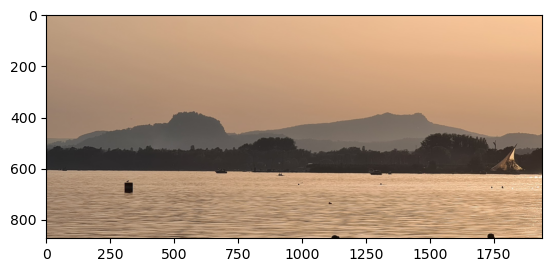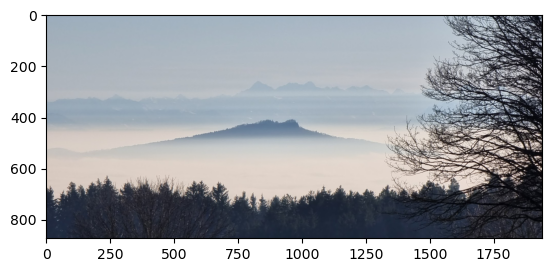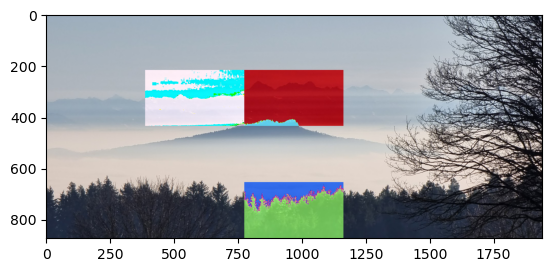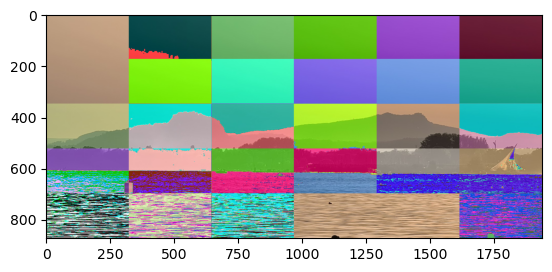# 23. Creating Videos from One or More Images

This chapter of our Python course deals with films and images. You will learn how to create films out of images.

## A Video from Multiple Pictures

So, you have a bunch of images and you would like to turn them into a vieo. In a way that each picture is shown for a certain amount of time. We assume that all your images, - which might be photographs you have taken in your last holiday or paintings you have made at same point in time - are in a directory. What we assume now is that all your paintings have the same size, if not you will also learn how to resize these images. For our purposes it might be good that the image names are following a special naming scheme, i.e. they all start with the same prefix (like 'pic_' e.g.) followed by a number (e.g. '001', '002', '003', ...) in an increasing order. The last part of the name is of course the suffix, which has to match the actual picture format.

You can download all the necessary images and the created videos for personal usage (copyright is by me) at python-courses: Material

First we provide a function 'seq_renaming' which will rename all the pictures in a folder according to the previously described naming scheme:

import glob
import os

def seq_renaming(folder='.', prefix='pic_', start=0, num_length=3):
count = start
folder += '/'
for fname in glob.glob(folder + '*.jpg'):
suffix = fname[fname.rfind('.'):]
outstr = f"{folder}/{prefix}{count:0{num_length}d}{suffix}"
count += 1
os.rename(fname, outstr)

seq_renaming('flower_images')


To test our newly created function and to demonstrate how it works, we create a test directory with empty files, simulating images:

import shutil
target_dir = 'test_dir'

if os.path.exists(target_dir):
# delete the existing directory
shutil.rmtree(target_dir)
# Create target_dir, because it doesn't exist so far
os.makedirs(target_dir)

names = ['apples', 'oranges', 'bananas', 'pears']
for name in names:
open(f'{target_dir}/{name}.jpg', 'w').write(' ')

print('Before renaming: ', os.listdir(target_dir))

# Let's rename the image names:
seq_renaming(target_dir)
print('After renaming: ', os.listdir(target_dir))


### OUTPUT:

Before renaming:  ['apples.jpg', 'oranges.jpg', 'bananas.jpg', 'pears.jpg']
After renaming:  ['pic_000.jpg', 'pic_001.jpg', 'pic_002.jpg', 'pic_003.jpg']


Our first video will be created by using the images in the folder flower_images. Let's look at one of the images.

import matplotlib.pyplot as plt
import random
import numpy as np
import cv2

plt.imshow(image)
#cv2.imshow(image)


### OUTPUT:

<matplotlib.image.AxesImage at 0x7f9793bbfbd0>The picture is taken in Radolfzell part of Lake Constance and in the background you can see the mountains Hohentwiel and Hohenstoffeln.

import matplotlib.pyplot as plt
import random
import numpy as np
import os
import cv2
import glob

def get_frame_size(image_path):
""" Reads an image and calculates
the width and length of the images,
which will be returned """
height, width, layers = frame.shape
frame_size = (width, height)
return frame_size

def video_from_images(folder='.',
video_name = 'video.avi',
suffix='png',
prefix='pic_',
reverse=False,
length_of_video_in_seconds=None,
codec = cv2.VideoWriter_fourcc(*'DIVX')):
""" The function creates a video from all the images with
the suffix (default is 'png' and the prefix (default is 'pic_'
in the folder 'folder'. If 'length_of_video_in_seconds' is set
to None, it will be the number of images in seconds. If a positive
value is given this will be the length of the video in seconds.
The function assumes that the the shape of the first image is
the one for all the images. If not a warning will be printed
and the size will be adapted accordingly! """

images = []
for fname in glob.glob(f'{folder}/{prefix}*{suffix}'):
images.append(fname)
images.sort(reverse=reverse)
if length_of_video_in_seconds is None:
# each image will be shown for one second
length_of_video_in_seconds = len(images)

# calculate number of frames per seconds:
frames_per_second = len(images) / length_of_video_in_seconds
frame_size = get_frame_size(images)

video = cv2.VideoWriter(video_name,
codec,
frames_per_second,
frame_size)

for image in images:
height, width, layers = im.shape
if (width, height) != frame_size:
print(f'Warning: {image} resized from {(width, height)} to {frame_size}')
im = cv2.resize(im, frame_size)
video.write(im)
cv2.destroyAllWindows()
video.release()

video_from_images('im2video/flower_images', 'flowers.avi', 'jpg')


### OUTPUT:

Warning: im2video/flower_images/pic_004.jpg resized from (3993, 1860) to (4000, 1800)
Warning: im2video/flower_images/pic_005.jpg resized from (4000, 2337) to (4000, 1800)


The result can be seen in flowers.avi!

Live Python training

Enjoying this page? We offer live Python training courses covering the content of this site.

Enrol here

## A Video from One Picture

We use now one picture as a basis to create a sequence of images. We do this by changing the colors of a part (a tile) of the image. We can think of the image as a checkerboard with n rows and m columns. The function random_tile can be used to return the top_left and bottom_right corner of a randomly chosen tile. We pass the image to the parameter 'img' and the number of rows and columns are defined by height_factor, width_factor:

import random

def random_tile(img, height_factor, width_factor):
""" returns the top left and bottom right corner
of a random tile in an image. The tile sizes are
determined by height_factor, width_factor, the division
factors """
height, width, colours = img.shape
tiles = height_factor, width_factor
tile_height, tile_width = height / height_factor, width / width_factor
tile_upper_left_row = int(round(random.randint(0, height_factor) * tile_height, 0))
tile_upper_left_column = int(round(random.randint(0, width_factor) * tile_width, 0))
top_left = tile_upper_left_row, tile_upper_left_column
bottom_right_row = int(round(tile_upper_left_row + tile_height, 0))
bottom_right_column = int(round(tile_upper_left_column + tile_width, 0))
bottom_right = bottom_right_row, bottom_right_column


In the following code, we read in an image and call the function random_tile a few times:

import matplotlib.pyplot as plt
import random
import numpy as np
print(f'{imag.shape=}')
for i in range(4):
tile = random_tile(imag, 4, 5)
print(tile)


### OUTPUT:

imag.shape=(873, 1941, 3)
((218, 1165), (436, 1553))
((436, 388), (654, 776))
((218, 776), (436, 1164))
((218, 1941), (436, 2329))


The function 'colorize_tile' can be used to change the color of a tile randomly. We do this by adding a random color value to each pixel of the tile.

def colorize_tile(imag, top_left, bottom_right, max_col_val):
color = np.random.randint(0, max_col_val, (3,))
r1, r2 = top_left, bottom_right
c1, c2 = top_left, bottom_right
imag[r1:r2, c1:c2] = imag[r1:r2, c1:c2] + color


We demonstrate the way of working of colorize_tile in the following code. Let's have a look at the images first. We use plt.imread instead of cv2.imread, because cv2.imshow opens an external window in the Jupyter-Notebook, which we use to create this website. Besides this the images of cv2.imread are in BGR-Format, whereas plt.imread returns RGB-Images. This means they are not compatible.

import matplotlib.pyplot as plt
import random
import numpy as np
plt.imshow(imag)


### OUTPUT:

<matplotlib.image.AxesImage at 0x7f97939cd490>Let's experiment with colorize_tile now:

imag = cv2.imread("im2video/images/20230215_161843.jpg")
for i in range(6):
top_left, bottom_right = random_tile(imag, 4, 5)
colorize_tile(imag, top_left, bottom_right, max_col_val=100)

# Let's save the result in 'experiments.png'
cv2.imwrite('experiments.png', imag)

# due to Jupyter-Noebook restraints, we have to use plt
# for reading and viewing again:


### OUTPUT:

<matplotlib.image.AxesImage at 0x7f97938b4150>We will create now 100 images in a folder called 'video_pics'. The original picture will be saved under the name pic_101.png in this folder. The image with the name 'pic_001.png' is the one with the most changed tiles:

pics_dir='im2video/video_pics'
n = 100
fname = f"{pics_dir}/pic_{n+1:03d}.png"
cv2.imwrite(fname, im)
for i in range(n, 0, -1):
top_left, bottom_right = random_tile(im, 5, 6)
colorize_tile(im, top_left, bottom_right, 100)
fname = f"{pics_dir}/pic_{i:03d}.png"
cv2.imwrite(fname, im)



Let's look at the final image 'pic_001.png' of the created images:

plt.imshow(plt.imread(f'{pics_dir}/pic_001.png'))


### OUTPUT:

<matplotlib.image.AxesImage at 0x7f9793924150>We will use now our function video_from_images again to create a video with the name 'rand_squares.avi' out of these newly created images:

video_from_images(pics_dir,
'random_squares.avi',
'png',
length_of_video_in_seconds = 280)


## A Video with Tiles from Multiple Images

We will create now pictures where we use one start picture and randomly replace tile by the corresponding tile of other pictures. The function 'tile_from_image' replaces the content of a tile of an image1 with the content of the corresponding tile of another image 'image2'. Both images need to have the same shape:

def tile_from_image(image1, image2, top_left, bottom_right):
""" take a tile from image2 and replace the corresponding
area in image1 """
r1, r2 = top_left, bottom_right
c1, c2 = top_left, bottom_right
image1[r1:r2, c1:c2] = image2[r1:r2, c1:c2]


In the following code we use pictures from the Bodensee (Lake Constance) are:

images_dir = 'im2video/bodensee_area'
target_dir = 'video_pics_bodensee'
if not os.path.exists(target_dir):
# Create target_dir, because it doesn't exist so far
os.makedirs(target_dir)
n_of_images = 120
import random

images = []
for fname in glob.glob(f'{images_dir}/*.jpg'):
images.append(fname)

imags = [cv2.imread(fname) for fname in images]
start_image = imags
for i in range(n_of_images, 0, -1):
top_left, bottom_right = random_tile(start_image, 5, 6)
image2 = random.choice(imags)
tile_from_image(start_image, image2, top_left, bottom_right)
fname = f"{target_dir}/pic_{i:03d}.png"
cv2.imwrite(fname, start_image)

video_from_images('video_pics_bodensee',
'bodensee.avi',
'png',
length_of_video_in_seconds = 60)


I used this technique to create a video for a music composition of mine. The video can be found at YouTube:

The music is the same in both videos. A music piece for string orchestra and solo cello. I added the sound track with the Linux program kdenlive.

Live Python training

Enjoying this page? We offer live Python training courses covering the content of this site.

Upcoming online Courses

Enrol here